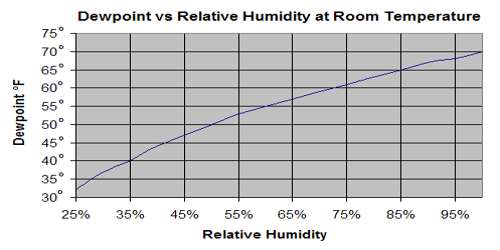Physics

# Relation between Dew Point and Relative HumidityRelation between dew point and relative humidity

From the definition of dew point we have already learnt that the temperature at which a given mass of air becomes just saturated by water vapour present in it is called its dew point. The dew point is the temperature at which a given sample of air will have a relative humidity of 100 percent; hence, the saturation temperature. On the other hand amount of saturation present in the atmosphere compared to the amount of water vapour present in the atmosphere is measured by relative humidity. It is the ratio (expressed as a percentage) of the amount of moisture actually in the air to the maximum amount that can be present at that temperature.

We can determine the relation between dew point and relative humidity by the help of Glaisher’s Theorem. Suppose the temperature at a place is θ1 and at that place temperature is θ2 for the wet thermometer bulb and dew point at that time is θ. So, dew point θ can be determined according to Glaisher’s theorem-

θ1 – θ = G (θ1 – θ2) …. …. (1)

Or, – θ = – θ1 + G (θ1 – θ2)

or, θ = θ1 – G (θ1 – θ2)

Here G = Glaisher’s factor or constant at θ1°C temperature. From Regnault’s table saturated water vapour pressure obtained at dew point θ°C = F.

According to the definition of relative humidity relative humity,

R = (Saturated vapour pressure at dew point) / (Saturated vapour pressure at air temperature)

R = f/F

If relative humidity is expressed in percentage, then,

R = f/F x 100%

This is the relation between dew point and relater humidity.